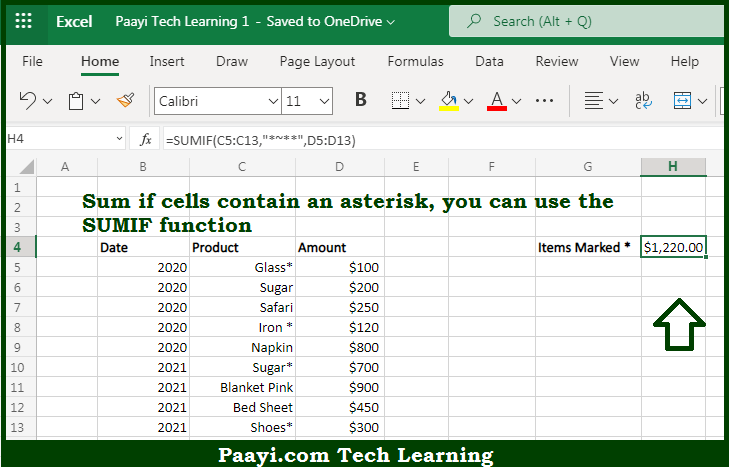# Learn How to SUM If Cells Contain An Asterisk in Microsoft Excel

Written by | 0 Comments | 573 Views

In this article, you will learn how to SUM various things in Microsoft Excel using a single/combination(s) of functions. You will also know how to SUM If Cells Contain An Asterisk and see the generic formula.

SUM If Cells Contain An Asterisk in Microsoft Excel

The main purpose of this formula is to sum if cells contain an asterisk. Here we will learn how to sum if the cell is having an asterisk in the workbook in Microsoft Excel. That implies, with the help of a formula based on the SUMIF function you can able to sum if cells contain an asterisk. So, with the help of this formula, you can able to sum if the cell is having an asterisk in the workbook in Microsoft Excel.

General Formula to SUM If Cells Contain An Asterisk

=SUMIF(range,"*~**",sum_range)

The Explanation for the SUM If Cells Contain An AsteriskSo we know that with the help of the given formula above you can able to sum if cells contain an asterisk. Here we will learn how to sum if the cell is having an asterisk in the workbook in Microsoft Excel. As we know that the SUMIF function fully supports wildcards. where the asterisk (*) means "one or more characters", while a question mark (?) means "any one character". With the help of these wildcards, you can able to create criteria such as "begins with", "ends with", "contains 3 characters", and so on. So, with the help of this formula, you can able to sum if the cell is having an asterisk in the workbook in Microsoft Excel.